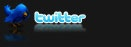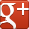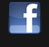top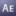# Wiggle, rubber, bounce, throw, inertia expressions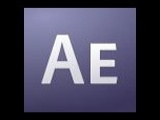These expressions save so much time, you might get home earlier tonight just by reading this.
After Effects CS3
Useful things
Expressions

Views: 999247
"If you've ever animated these sorts of things manually, you'll know what I mean. Most will need to be tweaked to your specific needs, but it's just a matter of playing with the numbers a little, and understanding how they'll change your animation."

Jumpy Wiggle 1 makes wiggle skip and hold rather than move fluidly.
``````// Jumpy Wiggle 1 (moves at a random FPS)
v=wiggle(5,50);
if(v < 50)v=0;
if(v > 50)v=100;
v``````
Jumpy Wiggle 2 is similar to 1, but works at a defined FPS so your "jump" will happen at a regular pace.
``````// Jumpy Wiggle 2 (moves at a defined FPS)
fps=5; //frequency
amount=50; //amplitude
wiggle(fps,amount,octaves = 1, amp_mult = 0.5,(Math.round(time*fps))/fps);``````
Inertial Bounce is like making your moves "rubbery." Layers will overextend, then settle into place on position and rotation keyframes.
``````// Inertial Bounce (moves settle into place after bouncing around a little)
n = 0;
if (numKeys > 0){
n = nearestKey(time).index;
if (key(n).time > time){
n--;
}
}
if (n == 0){
t = 0;
}else{
t = time - key(n).time;
}

if (n > 0){
v = velocityAtTime(key(n).time - thisComp.frameDuration/10);
amp = .05;
freq = 4.0;
decay = 2.0;
value + v*amp*Math.sin(freq*t*2*Math.PI)/Math.exp(decay*t);
}else{
value;
}``````
Sometimes you just want something to move constantly without keyframing it. Use throw.
``````// Throw (move at a constant speed without keyframes)
veloc = -10; //horizontal velocity (pixels per second)
x = position + (time - inPoint) *veloc;
y = position;
[x,y]``````
Same as throw, but for rotation.
``````// Spin (rotate at a constant speed without keyframes)
veloc = 360; //rotational velocity (degrees per second)
r = rotation + (time - inPoint) *veloc;
[r] ``````
by Chris Wright

(Min. Version: After Effects CS3, Category: Useful things, Type: Expressions)
posted by vfx007 on Oct 10, 2009

## Related topics:# Folding Template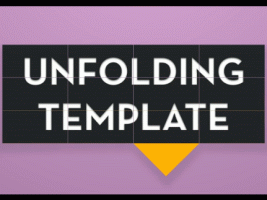folding and unfolding template free to use.
Require After Effects CS6
After Effects CS6
Other
Projects (aep)

Views: 53642
posted by fsimoni on Nov 05, 2012# Product, Service or App Promotion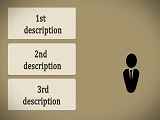After Effects Template. Promote your app, product or service
After Effects CS5
Useful things
Projects (aep)

Views: 29252
posted by RedSpikeFx on Oct 30, 2012# Clean Logo V2After Effects CS4,CS5,CS5.5 and CS6 template logo reveal
After Effects CS4
Other
Projects (aep)

Views: 34770
posted by aedaddy on Aug 19, 2012